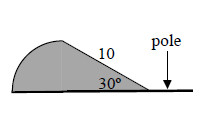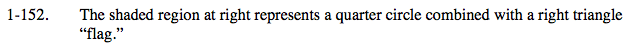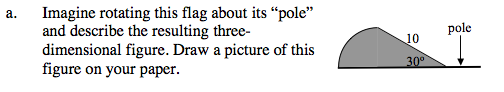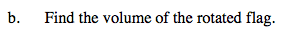### Home > CALC > Chapter 1 > Lesson 1.4.2 > Problem1-152

1-152.
1. The shaded region below represents a quarter circle combined with a right triangle "flag." Homework Help ✎1. Imagine rotating this flag about its "pole" and describe the resulting three-dimensional figure. Draw a picture of this figure on your paper. To help you visualize this, use the 1-152 eTool (Desmos).

2. Find the volume of the rotated flag.Use the eTool below to visualize the flag.
Click the link at right for the full version of the eTool: Calc 1-152 HW eToolSum together the volumes of the cone and the half-sphere. Note that the radius is obtained by using the trigonometry rule for 30° − 60° − 90° triangles.

$\text{The volume of a sphere is }\frac{4}{3}\pi r^{3},\text{ so the volume of half a}$

$\text{sphere would be half of that. The volume for a cone is }\frac{1}{3}\pi r^{2}h.$